Test Description

## 10 Questions MCQ Test Mathematics Olympiad for Class 1 | Olympiad Test Level 2: Addition- 2

 1 Crore+ students have signed up on EduRev. Have you?

### Bunny sells 4 oranges on Monday and 5 oranges on Tuesday. Nidhi sells 12 oranges on Monday and 5 oranges on Tuesday. How many oranges do they sell altogether on Monday and Tuesday?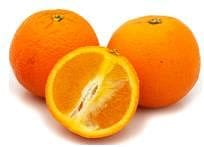Detailed Solution for Olympiad Test Level 2: Addition- 2 - Question 1

4 + 5 + 12 + 5 = 26

### Identify the correct option for the following: 23 + 25 =

Detailed Solution for Olympiad Test Level 2: Addition- 2 - Question 2

23 + 25 = 40 + 8 = 48

### If one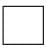represents 3, which one of the following options is true for 12?

Detailed Solution for Olympiad Test Level 2: Addition- 2 - Question 3

3 + 3 + 3 + 3 = 12

What is the sum of the first five counting numbers?

Detailed Solution for Olympiad Test Level 2: Addition- 2 - Question 4

1 + 2 + 3 + 4 + 5 = 15

The temperature in Delhi is 17°C. If the temperature in Pune is 8°C more than the temperature in Delhi, write the temperature in Pune.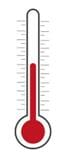Detailed Solution for Olympiad Test Level 2: Addition- 2 - Question 5

17 + 8 = 25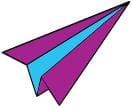denotes the number 4. What will be the number denoted by the below image?Detailed Solution for Olympiad Test Level 2: Addition- 2 - Question 6

4 + 4 + 4 + 4 = 16

Which number will you write in the box so that the results become the same?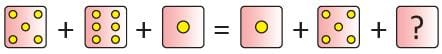Detailed Solution for Olympiad Test Level 2: Addition- 2 - Question 7

5 + 6 + 1 = 1 + 5 + 6 = 12

Seven numbers are given inside the star. What will be the number obtained by the sum of the smallest and the largest numbers?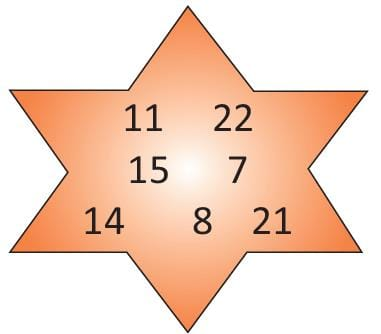Detailed Solution for Olympiad Test Level 2: Addition- 2 - Question 8

Largest number = 22 and smallest number = 7.
So, sum = 22 + 7 = 29

In a progress card, total marks have to be calculated for the two entries 15 and 32. If the student obtained 23 marks instead of 32, what will be the total of the student?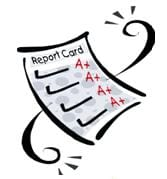Detailed Solution for Olympiad Test Level 2: Addition- 2 - Question 9

15 + 23 = 38

Sachin has 11 chocolates and Seema has 4 chocolates. If Sachin gives 8 chocolates to Seema, how many chocolates will Seema have now?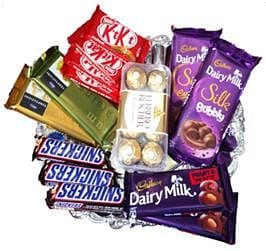Detailed Solution for Olympiad Test Level 2: Addition- 2 - Question 10

4 + 8 = 12

## Mathematics Olympiad for Class 1

9 docs|51 tests
 Use Code STAYHOME200 and get INR 200 additional OFF Use Coupon Code